# ISEE Upper Level Quantitative : How to find area

## Example Questions

### Example Question #10 : Other Polygons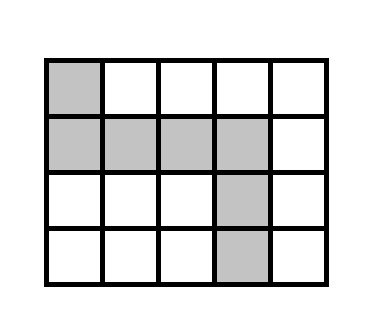The above rectangle, which has perimeter 360, is divided into squares of equal size. Give the area of the shaded portion.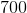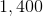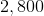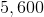Explanation:

The sides of the rectangle, in total, are divided into 18 segments of equal measure, as indicated below: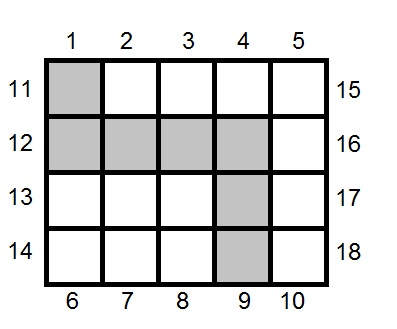The rectangle has a total perimeter of 360, so each segment - one side of a square - measures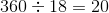. Each square has area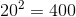, so the shaded portion of the rectangle, which comprises seven squares, has area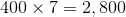.